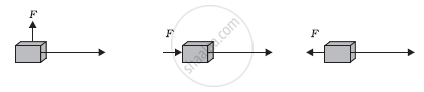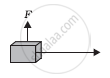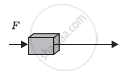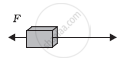Share

# In Each of the Following a Force, F Is Acting on an Object of Mass, M. the Direction of Displacement is from West to East Shown by the Longer Arrow. Observe the Diagrams Carefully and State Whether the Work Done by the Force is Negative, Positive Or Zero. - CBSE Class 9 - Science

ConceptWork Done by a Constant Force

#### Question

In each of the following a force, F is acting on an object of mass, m. The direction of displacement is from west to east shown by the longer arrow. Observe the diagrams carefully and state whether the work done by the force is negative, positive or zero.#### Solution

Work is done whenever the given two conditions are satisfied:

(i) A force acts on the body.

(ii) There is a displacement of the body by the application of force in or opposite to the direction of force.

Case IIn this case, the direction of force acting on the block is perpendicular to the displacement. Therefore, work done by force on the block will be zero.

Case IIIn this case, the direction of force acting on the block is in the direction of displacement. Therefore, work done by force on the block will be positive.

Case IIIIn this case, the direction of force acting on the block is opposite to the direction of displacement. Therefore, work done by force on the block will be negative.

Is there an error in this question or solution?

#### APPEARS IN

NCERT Solution for Science Textbook for Class 9 (2018 to Current)
Chapter 11: Work and Energy
Q: 18 | Page no. 159
Solution In Each of the Following a Force, F Is Acting on an Object of Mass, M. the Direction of Displacement is from West to East Shown by the Longer Arrow. Observe the Diagrams Carefully and State Whether the Work Done by the Force is Negative, Positive Or Zero. Concept: Work Done by a Constant Force.
S